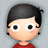#### 学习笔记TF048:TensorFlow 系统架构、设计理念、编程模型、API、作用域、批标准化、神经元函数优化

``````inport tensorflow as tf
#创建图
a = tf.constant([1.0,2.0])
b = tf.constant([3.0,4.0])
c = a * b
#计算c
print sess.run(c)#进行矩阵乘法，输出[3.,8.]
sess.close()
``````

``````import tensorflow as tf
#创建一个常量运算操作，产生一个1x2矩阵
matrix1 = tf.constant([[3.,3.]])
#创建另外一个常量运算操作，产生一个2x1矩阵
matrix2 = tf.constant([[2.],[2.]])
#创建一个矩阵乘法运算，把matrix1和matrix2作为输入
#返回值product代表矩阵乘法结果
product = tf.matmul(matrix2,matrix2)
``````

``````with tf.Session as sess:
result = sess.run([product])
print result
``````

``````#创建一个变量，初始化为标量0
state = tf.Variable(0,name="counter")
``````

``````state = tf.constant(3.0)
``````

``````input1 = tf.placeholder(tf.float32)
input2 = tf.placeholder(tf.float32)
output = tf.mul(input1,input2)
with tf.Session() as sess:
#输出[array([24.],dtype=float32)]
print sess.run([output],feed_dict={input1:[7.],input2:[2.]})
``````

tf.Operaiotn类代表图中节点，用于计算张量数据。由节点构造器(如tf.matmul()、Graph.createop())产生。 操作 描述 tf.Operation.name 操作名称 tf.Operation.type 操作类型 tf.Operation.inputs 操作输入 tf.Operation.outputs 操作输出 tf.Operation.controlinputs 操作依赖 tf.Operation.run(feeddict=None,session=None) 在会话中运行操作 tf.Operation.getattr(name) 获取操作属性值

tf.Tensor类，操作输出符号句柄，不包含操作输出值，提供在tf.Session中计算值方法。操作间构建数据流连接，TensorFlow能免执行大量步多计算图形。 操作 描述 tf.Tensor.dtype 张量数据类型 tf.Tensor.name 张量名称 tf.Tensor.valueindex 张量操作输出索引 tf.Tensor.graph 张量所在图 tf.Tensor.op 产生张量操作 tf.Tensor.consumers() 返回使用张量操作列表 tf.Tensor.eval(feeddict=None,session=None) 会话中求张量值，使用sess.asdefault()、eval(session=sess) tf.Tensor.getshape() 返回表示张量形状(维度)类TensorShape tf.Tensor.set_shape(shape) 更新张量形状 tf.Tensor.device 设置计算张量设备

``````#计算Wx_plus_b均值方差，axes=标准化维度
fc_mean,fc_var = tf.nn.moments(Wx_plus_b, axes=)
scale = tf.Variable(tf.ones([out_size]))
shift = tf.Variable(tf.zeros([out_size]))
epsilon = 0.001
Wx_plus_b = tf.nn.batch.normalization(Wx_plus_b,fc_mean,fc_var,shift,scale,epsilon)
#Wx_plus_b = (Wx_plus_b - fc_mean) / tf.sqrt(fc_var + 0.001)
#Wx_plus_b = Wx_plus_b * scale + shift
``````

《Batch Normalization:Accelerating Deep Network Training by Reducing Internal Covariate Shift》，Serger Ioffe、Christian Szegedy。